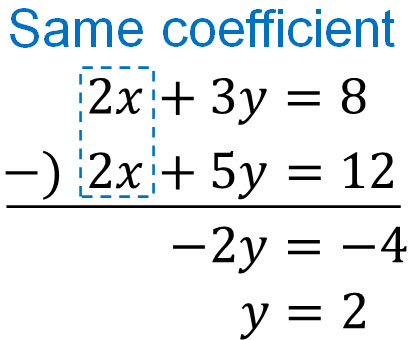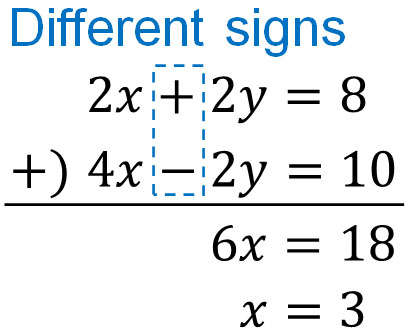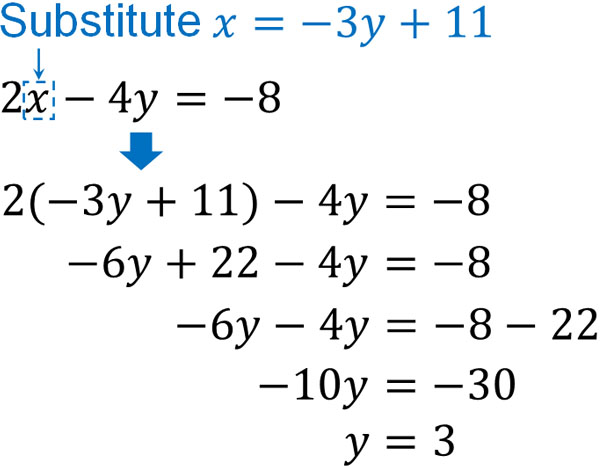In mathematics, we may use two equations to solve a calculation problem. If there are two unknown numbers in the equation, we cannot find the answer. However, if there are two equations, we can find the answer even if there are two unknown numbers in the equation.

A combination of two or more equations is called simultaneous equations (or a system of linear equations). By using a system of linear equations, we can get an answer even if there is more than one unknown answer.

There are two ways to solve simultaneous equations. Also, mathematics is used in everyday life, and it is important to be able to solve word problems in simultaneous equations. In other words, you need to not only learn the definition and how to solve simultaneous equations, but you also need to be able to create equations and solve problems on your own.

Therefore, we will explain not only how to solve simultaneous equations, but also how to create equations in word problems.

## There Are Two Ways to Solve a System of Linear Equations

The linear equation you learn in mathematics is the following equation.

• $2x+3y=8$

However, this equation has an infinite number of answers. This is because the values of $x$ and $y$ can be changed at will. There are many patterns of $x$ and $y$ that make the answer 8.

So, in simultaneous equations, we use two or more expressions. For example, we have the following.

$\begin{eqnarray} \left\{\begin{array}{l}2x+3y=8\\2x+5y=12\end{array}\right.\end{eqnarray}$

There is one $x$ and one $y$ that satisfy both equations. Even if there are multiple unknown numbers, you can get a specific answer by using simultaneous equations. It is important to note that there are two ways to solve simultaneous equations. The two methods are as follows.

• Substitution method

We will explain each of these methods.

## Addition/Subtraction Method: Remove $x$ or $y$ by Addition or Subtraction

The method of solving a system of equations by addition or subtraction is called the addition/subtraction method. By adding or subtracting a linear equation, you can eliminate either $x$ or $y$.

For example, in the case of the simultaneous equations described above, the common letter is $2x$. So, by subtracting, we can make it into a linear equation, as shown below.If the coefficients are the same, the letters can be eliminated by the addition/subtraction method. In this calculation, we can find the answer $y=2$ by subtracting the equations from each other.

-Substitute and Get the Value of $x$ or $y$

Then, get the other answer. Now that we know $y=2$, we can find the value of $x$. Substitute $y=2$ for either of the following expressions.

$\begin{eqnarray} \left\{\begin{array}{l}2x+3y=8\\2x+5y=12\end{array}\right.\end{eqnarray}$

You can substitute $y=2$ into either one. Both will give the same answer.

• For $2x+3y=8$

$2x+3×2=8$

$2x+6=8$

$2x=2$

$x=1$

• For $2x+5y=12$

$2x+5×2=12$

$2x+10=12$

$2x=2$

$x=1$

It is a system of linear equations that give us $x$ and $y$ that satisfy the two equations. So, naturally, the final answer is the same no matter which equation you substitute in.

### Distinguish Addition and Subtraction with Plus and Minus

To determine whether you should add or subtract, check the sign. Even if the absolute values of the coefficients are the same, the calculation method will change depending on whether the sign is positive or negative.

In the previous system of linear equations, the absolute value of the coefficients and the signs are the same. Therefore, we subtracted it. On the other hand, what if the absolute values of the coefficients are the same, but the signs are different? For example, we have the following case.

$\begin{eqnarray} \left\{\begin{array}{l}2x+2y=8\\4x-2y=10\end{array}\right.\end{eqnarray}$

In this case, do the addition. It looks like the following.You can then substitute $x=3$ to get the answer $y=1$.

As for whether you add or subtract in the addition/subtraction method, make a distinction depending on whether the letter you want to eliminate is positive or negative.

### Align the Coefficients of $x$ or $y$

Earlier, we used simple examples with simultaneous equations to explain them. However, in actual calculations, the absolute values of $x$ and $y$ in each equation are often different. For example, what is the answer to the following simultaneous equations?

$\begin{eqnarray} \left\{\begin{array}{l}2x+3y=16\\3x-4y=10\end{array}\right.\end{eqnarray}$

Both $x$ and $y$ have different absolute values. So try to match the absolute values by multiplying both sides. It is as follows.In this way, we can align the absolute values of the numbers in front of the letters. We can then add or subtract the equation to get the values of $x$ and $y$.

It is up to you to decide which of the absolute values of $x$ and $y$ you want to align with. Try to match the absolute values of the coefficients to make the calculation easier.

-When Calculating Fractions, the Basic Rule Is to Eliminate Fractions

In a system of equations, there are often fractions in the equation. Adding and subtracting fractions is difficult and often leads to miscalculation.

Therefore, try to eliminate fractions by multiplying both sides of the equation. When fractions are in simultaneous equations, the trick is to eliminate the fractions to get the answer.

## Eliminate Letters by Substitution Method

In a system of equations, there is one more way to solve simultaneous equations. That is the substitution method. When solving a problem, you must have substituted a specific number into $x$ or $y$ to get an answer. In mathematics, substitution can be done not only with numbers but also with letters.

So in a system of linear equations, you can get the answer by substituting. For example, let’s solve the following simultaneous equations using the substitution method.

$\begin{eqnarray} \left\{\begin{array}{l}x+3y=11\\2x-4y=-8\end{array}\right.\end{eqnarray}$

In the substitution method, it must be an expression of $x=□$ or $y=□$. In addition, the coefficient in front of the letter must be 1.

In the previous simultaneous equation, change the form of $x+3y=11$. We can convert it to the form $x=□$ by transposing $3y$, as shown below.

$x+3y=11$

$x=-3y+11$

By changing the form of the equation, we get $x=-3y+11$. Since $x$ is $-3y+11$, we can substitute it into the other equation, $2x-4y=-8$. Then we can eliminate $x$ just as we did with the addition method.And by substituting $y=3$ into the equation, we get the answer $x=2$.

$x=-3y+11$

$x=-3×3+11$

$x=2$

In the substitution method, set the coefficient of $x$ or $y$ to 1. After creating the equation $x=□$ or $y=□$, the answer can be found by substituting the other equation.

### Either Addition or Substitution Method Can Be Used

Some people wonder whether they should use the addition method or the substitution method when solving a system of equations. For this, you can use either method.

Depending on the problem, it may be easier to use the addition/subtraction method. And sometimes, it is easier to use the substitution method for some calculations. In mathematics, the simpler the calculation, the fewer mistakes you will make. Therefore, you should be able to perform both the addition/subtraction method and substitution method.

What is important is the fact that there are two ways to answer a system of linear equations.

Which is the best method to solve depends on the problem. So, solve the simultaneous equations by considering which one is easier to calculate.

## How to Solve Word Problems in a System of Equations

After you understand how to solve simultaneous equations, you have to be able to answer word problems.

Before learning a system of linear equations, we always learn linear equations. In linear equations, we must also solve word problems. There is a certain way to solve word problems in mathematics, and you have to create an equation from the problem sentence. Even with simultaneous equations, you have to create the equations first.

In linear equations, the unknown number is $x$. In simultaneous equations, on the other hand, there are two unknown numbers. So set the unknown numbers to $x$ and $y$, respectively. We then create a system of linear equations to find the answer. The whole process is as follows.

• Set the unknown numbers to $x$ and $y$.
• Create two equations.
• Solve simultaneous equations.

In algebraic expressions, we always make unknown numbers into the alphabet. So use $x$ and $y$ to create expressions.

### Example of a Word Problem Using Simultaneous Equations

Now, let’s try to solve a text problem using simultaneous equations.

Q: Solve the following word problem.

You bought oranges at \$2 each and watermelons at \$5 each for a total of 20 oranges and watermelons, and the total is \$82. How many oranges and watermelons did you buy, respectively? A: Answer. In word problems in a system of equations, the unknown numbers are$x$and$y$. The unknown numbers are the number of oranges and watermelons you bought. So, we do the following. • The number of oranges bought:$x$• The number of watermelons bought:$y$Then we can make the following two expressions.$\begin{eqnarray} \left\{\begin{array}{l}x+y=20\\2x+5y=82\end{array}\right.\end{eqnarray}$The total number of oranges and watermelons is 20. Therefore,$x+y=20$. Also, the amount of oranges is$2×x$. The amount of watermelon is$5×y$. The total amount is \$82, so it must be $2x+5y=82$. Then, we solve this system of linear equations. If we use the substitution method, we do the following.

$x+y=20$

$x=20-y$

Next, we substitute $x=20-y$.

$2\textcolor{red}{(20-y)}+5y=82$

$40-2y+5y=82$

$3y=42$

$y=14$

And by substituting $y=14$, we get $x=6$. So we can see that you bought 6 oranges and 14 watermelons.

### Be Sure to Substitute and Review the Answer

When solving simultaneous equations, you should always review your answers after you get them. Reviewing is the key to reducing miscalculations when solving problems.

For example, solve the following system of equations.

$\begin{eqnarray} \left\{\begin{array}{l}4x+3y=14\\3x+2y=11\end{array}\right.\end{eqnarray}$

For example, assume that we solve this problem and get $x=3,y=1$. But is this answer really correct? Let’s apply it to both equations, not just one.

• Calculate $4x+3y=14$.

$4×3+3×1=15$ : Wrong

• Calculate $3x+2y=11$

$3×3+2×1=11$ : Correct

As you can see, the answer does not match in one equation. So we know that the calculation is wrong because the answer is the one that satisfies the two equations.

Recalculating, we get $x=5,y=-2$. In this case, the answer satisfies both equations. Everyone makes a miscalculation. However, we can prevent miscalculations by reviewing them.

## Exercises: How to Solve Simultaneous Equations and Word Problems

Q1: Solve the following simultaneous equations.

(a)

$\begin{eqnarray} \left\{\begin{array}{l}0.4x+0.8y=6\\2x+1.2y=16\end{array}\right.\end{eqnarray}$

(b)

$\begin{eqnarray} \left\{\begin{array}{l}\displaystyle\frac{2}{3}x-\displaystyle\frac{3}{4}y=-5\\-\displaystyle\frac{1}{6}x+\displaystyle\frac{4}{2}y=23\end{array}\right.\end{eqnarray}$

If fractions are included in the equation, eliminate them by multiplying both sides of the equation. In addition, you should align the absolute values.

(a)

$\begin{eqnarray} \left\{\begin{array}{l}0.4x+0.8y=6\\2x+1.2y=16\end{array}\right.\end{eqnarray}$

Checking $x$ and $y$, it seems easier to match the coefficients of $x$. So, we do the following.

$0.4x+0.8y=6$

$(0.4x+0.8y)\textcolor{red}{×5}=6\textcolor{red}{×5}$

$2x+4y=30$

Therefore, it can be converted to the following simultaneous equation.

$\begin{eqnarray} \left\{\begin{array}{l}2x+4y=30\\2x+1.2y=16\end{array}\right.\end{eqnarray}$

Calculating this is as follows.

$\begin{array}{r}2x+4y=30\\\underline{-)\phantom{0}2x+1.2y=16}\\2.8y=14\end{array}$

Calculating $2.8y=14$, we get $y=5$. Also, by substituting $y=5$ into the system of equations, we get $x=5$. Therefore, $x=5,y=5$ is the correct answer.

(b)

$\begin{eqnarray} \left\{\begin{array}{l}\displaystyle\frac{2}{3}x-\displaystyle\frac{3}{4}y=-5\\-\displaystyle\frac{1}{6}x+\displaystyle\frac{4}{2}y=23\end{array}\right.\end{eqnarray}$

We can solve the problem by either addition method or substitution method. We can also eliminate fractions by multiplication of both sides. Fractions in each equation can be eliminated by the following calculation.

• $\displaystyle\frac{2}{3}x-\displaystyle\frac{3}{4}y=-5$

$\left(\displaystyle\frac{2}{3}x-\displaystyle\frac{3}{4}y\right)\textcolor{red}{×12}=-5\textcolor{red}{×12}$

$8x-9y=-60$

• $-\displaystyle\frac{1}{6}x+\displaystyle\frac{4}{2}y=23$

$\left(-\displaystyle\frac{1}{6}x+\displaystyle\frac{4}{2}y\right)\textcolor{red}{×(-6)}=23\textcolor{red}{×(-6)}$

$x-12y=-138$

$x=12y-138$

Then substitute $x=12y-138$. In this example, we solve the problem by substitution method, not by addition/subtraction method.

$8\textcolor{red}{(12y-138)}-9y=-60$

$96y-1104-9y=-60$

$87y=1044$

$y=12$

Substituting $y=12$ to get the answer, we get $x=6,y=12$.

Q2: Solve the following word problem.

Leaving the house, you headed to the library, which is 2400 meters away. At first, you ran at 100 m/min, but you got tired on the way and walked at 40 m/min. It took you 30 minutes to reach the library. Find the time you ran and the time you walked.

Set the time spent running to $x$ minutes and the time spent walking to $y$ minutes. Since the total time of running and walking is 30 minutes, the following equation holds.

• $x+y=30$

Also, the distance ran is $100×x$. In contrast, the distance walked is $40×y$. Since it is 2400 meters from the house to the library, the following equation holds.

• $100x+40y=2400$

Next, solve the following simultaneous equations.

$\begin{eqnarray} \left\{\begin{array}{l}x+y=30\\100x+40y=2400\end{array}\right.\end{eqnarray}$

Solved by addition method, the result is as follows.

$x+y=30$

$(x+y)\textcolor{red}{×40}=30\textcolor{red}{×40}$

$40x+40y=1200$

$\begin{array}{r}40x+40y=1200\\\underline{-)\phantom{0}100x+40y=2400}\\-60x=-1200\end{array}$

Calculating $-60x=-1200$, we get $x=20$. Substituting $x=20$ then gives $y=10$. Therefore, the time spent running is 20 minutes and the time spent walking is 10 minutes.

## Solving Simultaneous Equations by Addition/Subtraction and Substitution Methods

By using a system of linear equations, we can get the answer even if we have two unknown numbers. There are two ways to do this: the addition/subtraction method and the substitution method. In a system of equations, both methods are used. So, make sure you choose the best method.

Furthermore, there are often problems involving fractions. Even if there are fractions, the solution is the same. Multiply both sides of the equation and align the coefficients.

Another important thing in mathematics is to be able to solve word problems. Being able to create an equation allows you to apply math in your daily life. Making two equations is important when solving word problems.